### New style dragon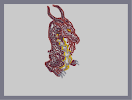Hover over the thumbnail for a full-size version.

Author Dragon_Moon author:dragon_moon n-art rated 2006-01-28 4 by 48 people. \$New style dragon#Dragon_Moon#none#00000000000000000000000000000000000000000000000000000000000000000000000000000000000000000000000000000000000000000000000000000000000000000000000000000000000000000000000000000000000000000000000000000000000000000000000000000000000000000000000000000000000000000000000000000000000000000000000000000000000000000000000000000000000000000000000000000000000000000000000000000000000000000000000000000000000000000000000000000000000000000000000000000000000000000000000000000000000000000000000000000000000000000000000000000000000000000000000000000000000000000000000000000000000000000000000000000000000000000000000000000000000000000000000000000000000000000000000000000000000000000000000000000000000000000000000000000000000000000|12^463,260!12^463,255!12^462,248!12^457,243!12^450,239!12^445,239!12^439,239!12^433,239!12^433,235!12^430,228!12^425,223!12^418,221!12^409,221!12^412,221!12^403,223!12^403,216!12^401,213!12^397,212!12^390,212!12^390,207!12^390,203!12^387,200!12^384,196!12^381,192!12^380,189!12^461,262!12^467,257!12^471,252!12^474,246!12^475,242!12^475,237!12^473,231!12^469,225!12^464,219!12^465,221!12^458,214!12^453,212!12^447,211!12^444,206!12^441,202!12^440,197!12^436,192!12^436,193!12^438,187!12^438,184!12^435,179!12^429,173!12^423,167!12^425,169!12^430,193!12^426,192!12^425,188!12^425,186!12^423,180!12^421,177!12^418,175!12^412,173!12^407,173!12^402,171!12^398,167!12^441,191!12^446,189!12^448,184!12^448,179!12^445,175!12^441,170!12^438,165!12^436,161!12^447,192!12^448,198!12^451,203!12^455,207!12^459,210!12^402,175!12^405,181!12^409,187!12^414,190!12^418,191!12^454,242!12^461,213!12^472,227!12^476,244!12^462,229!12^467,234!12^467,239!12^457,252!12^453,253!12^447,253!12^441,253!12^433,250!12^430,249!12^425,245!12^423,242!12^420,238!12^415,233!12^437,252!12^412,231!12^408,229!12^398,225!12^402,227!12^392,224!12^387,224!12^381,224!12^380,221!12^378,219!12^373,217!12^371,217!12^369,215!12^369,212!12^369,210!12^369,207!12^369,205!12^367,204!12^365,203!12^365,201!12^366,199!12^368,196!12^368,193!12^365,190!12^364,188!12^365,186!12^369,184!12^371,180!12^371,178!12^371,176!12^416,236!12^378,226!12^375,229!12^375,233!12^375,238!12^375,241!12^375,245!12^375,249!12^376,251!12^377,252!12^368,220!12^366,223!12^366,228!12^366,235!12^367,239!12^367,242!12^371,245!12^371,248!12^373,250!12^365,211!12^361,212!12^358,216!12^355,221!12^355,228!12^355,232!12^355,235!12^351,228!12^351,222!12^351,215!12^352,210!12^356,202!12^360,200!12^353,205!12^351,231!12^360,189!12^356,189!12^352,189!12^347,189!12^344,191!12^341,193!12^338,197!12^337,201!12^337,203!12^338,204!12^342,200!12^344,200!12^348,199!12^354,199!12^356,199!12^368,174!12^366,171!12^360,168!12^355,168!12^350,168!12^344,168!12^341,170!12^347,170!12^351,172!12^356,175!12^357,177!12^357,180!12^359,183!12^441,257!12^436,261!12^435,265!12^436,270!12^439,274!12^441,279!12^442,291!12^442,297!12^441,304!12^437,313!12^433,316!12^442,285!12^438,311!12^431,257!12^428,260!12^428,267!12^430,274!12^432,280!12^432,289!12^432,300!12^430,305!12^426,312!12^430,293!12^431,283!12^425,315!12^425,321!12^427,325!12^430,329!12^425,249!12^425,253!12^424,265!12^424,270!12^423,278!12^422,282!12^420,285!12^418,289!12^418,294!12^418,298!12^417,243!12^417,248!12^416,255!12^416,259!12^416,266!12^412,271!12^408,236!12^408,241!12^408,247!12^408,251!12^405,254!12^448,258!12^447,262!12^447,270!12^448,276!12^449,280!12^449,286!12^449,295!12^447,266!12^451,292!0^408,176!0^411,178!0^417,182!0^412,181!0^414,184!0^420,187!12^413,174!12^411,191!12^412,189!12^419,191!12^377,146!12^379,142!12^380,140!12^383,138!12^386,136!12^389,135!12^393,135!12^400,140!12^397,138!12^404,145!12^408,148!12^411,152!12^415,155!12^403,134!12^427,140!12^430,146!12^433,150!12^433,153!12^436,157!12^406,130!12^411,130!12^414,130!12^418,131!12^423,136!12^387,131!12^384,128!12^382,125!12^377,122!12^370,118!12^367,116!12^373,121!12^363,114!12^362,113!12^356,113!12^353,112!12^346,111!12^338,109!12^334,108!12^330,107!12^326,104!12^321,100!12^314,95!12^309,91!12^305,87!12^302,83!12^300,79!12^296,69!12^296,51!12^296,54!12^296,60!12^296,65!12^296,70!12^298,74!12^298,77!12^294,48!12^294,45!12^291,42!12^288,39!12^286,38!12^286,38!12^286,41!12^288,45!12^288,50!12^288,58!12^288,64!12^288,69!12^288,75!12^288,80!12^291,86!12^292,88!12^294,92!12^298,96!12^301,99!12^303,101!12^344,122!12^349,125!12^353,127!12^362,132!12^366,136!12^373,142!12^376,146!12^370,139!12^368,137!12^357,130!12^289,55!12^306,105!12^309,108!12^314,110!12^317,113!12^322,116!12^327,119!12^339,122!12^332,121!12^341,110!12^410,125!12^409,124!12^407,120!12^404,118!12^401,116!12^398,114!12^394,113!12^392,112!12^390,110!12^387,106!12^384,101!12^384,97!12^383,92!12^382,88!12^382,82!12^381,76!12^380,71!12^377,67!12^374,62!12^371,58!12^366,52!12^363,48!12^359,45!12^354,40!12^347,35!12^341,34!12^334,34!12^326,32!12^319,30!12^331,35!12^319,30!12^318,31!12^319,33!12^320,34!12^321,35!12^326,37!12^329,38!12^334,40!12^337,40!12^342,42!12^345,44!12^346,45!12^350,37!12^350,48!12^354,52!12^358,56!12^360,61!12^363,65!12^366,71!12^368,76!12^370,83!12^370,89!12^371,95!12^371,100!12^373,107!12^376,114!12^378,120!12^368,56!12^369,108!12^360,108!12^353,109!12^363,108!12^379,113!12^385,116!12^387,117!12^392,123!12^397,131!12^392,125!12^385,119!12^390,121!12^396,129!12^325,123!12^320,125!12^317,128!12^312,131!12^306,136!12^303,141!12^301,145!12^300,152!12^299,157!12^297,163!12^296,169!12^294,174!12^294,181!12^294,191!12^294,199!12^294,207!12^294,215!12^294,221!12^296,229!12^297,234!12^301,240!12^307,250!12^310,259!12^311,265!12^312,271!12^313,277!12^313,283!12^313,289!12^311,296!12^309,300!12^303,303!12^298,306!12^293,306!12^291,306!12^308,256!12^303,245!12^302,243!12^293,182!12^293,189!12^293,200!12^293,207!12^293,212!12^293,194!12^308,133!12^294,182!12^293,224!0^381,229!0^381,232!0^382,239!0^384,243!0^385,245!0^387,247!0^390,250!0^393,251!0^394,253!0^389,258!0^389,267!0^388,261!0^389,271!0^390,274!0^392,277!0^391,278!0^388,278!0^384,277!0^379,276!0^374,274!0^370,273!0^364,273!0^358,273!0^355,273!0^356,271!0^357,265!0^357,262!0^357,258!0^356,253!0^354,249!0^365,251!0^361,249!0^354,246!0^349,246!0^343,246!0^343,245!0^346,239!0^348,236!0^345,221!0^340,220!0^338,219!0^336,218!0^337,217!0^342,214!0^344,209!0^345,205!0^372,280!0^374,283!0^374,286!0^374,292!0^371,297!0^368,299!0^369,299!0^375,299!0^379,300!0^382,301!0^384,302!0^388,306!0^394,308!0^397,310!0^403,315!0^403,317!0^403,319!0^403,314!0^403,308!0^403,300!0^403,294!0^405,286!0^406,282!0^409,278!0^410,276!0^407,276!0^402,272!0^398,267!0^392,263!0^405,275!0^397,267!0^388,309!0^388,316!0^388,321!0^387,326!0^382,330!0^378,332!0^380,333!0^386,334!0^394,337!0^403,342!0^407,347!0^407,351!0^399,340!0^410,369!0^414,348!0^417,340!0^418,336!0^420,333!0^424,330!0^418,306!0^414,304!0^410,299!0^422,309!0^389,335!0^419,344!12^371,154!12^367,150!12^364,147!12^360,145!12^357,143!12^352,141!12^348,140!12^343,137!12^338,134!12^334,133!12^330,133!12^325,133!12^325,136!12^329,137!12^332,138!12^336,139!12^338,139!12^343,141!12^346,142!12^355,144!12^352,145!12^333,144!12^338,149!12^342,150!12^349,154!12^356,155!12^360,159!12^363,163!12^341,150!3^340,151!3^346,152!12^341,315!12^345,316!12^349,319!12^356,324!12^359,327!12^362,335!12^362,340!12^362,347!12^362,356!12^361,366!12^358,369!12^353,371!12^361,359!12^362,331!12^300,328!12^297,330!12^297,337!12^297,343!12^299,345!12^300,351!12^303,354!12^305,356!12^310,361!12^361,353!12^362,371!12^359,376!12^357,383!12^355,386!12^350,390!12^345,394!12^342,398!12^336,402!12^332,403!12^326,405!12^319,405!12^317,403!12^314,403!12^310,401!12^306,399!12^304,398!12^299,395!12^296,393!12^304,361!12^300,363!12^292,367!12^291,368!12^287,370!12^283,373!12^279,373!12^277,373!12^274,376!12^272,379!12^272,385!12^276,391!12^277,394!12^284,400!12^289,408!12^287,406!12^279,398!12^291,413!12^294,415!12^296,417!12^297,420!12^300,422!12^304,424!12^310,428!12^314,428!12^320,430!12^323,434!12^328,438!12^331,442!12^336,447!12^340,449!12^342,451!12^342,453!12^344,454!12^334,445!12^348,456!12^351,456!12^354,456!12^356,455!12^355,440!12^353,438!12^351,438!12^349,438!12^345,435!12^341,430!12^338,427!12^336,424!12^340,414!12^343,418!12^345,419!12^351,423!12^353,425!12^357,426!12^358,429!12^362,432!12^363,433!12^366,435!12^356,453!12^351,451!12^349,445!2^357,450,0,-1!2^357,450,0.707106781186547,-0.707106781186547!2^366,454,0.707106781186547,-0.707106781186547!2^370,461,1,0!2^355,456,0,-1!2^364,457,0.707106781186547,-0.707106781186547!2^365,440,0,-1!2^372,445,0,-1!2^371,426,0,-1!2^373,430,0,-1!2^382,427,0,-1!12^341,401!12^344,402!12^346,405!12^348,405!12^353,407!12^358,411!12^361,411!12^365,417!12^370,420!12^373,420!0^394,341!0^394,347!0^394,351!0^393,355!0^383,359!0^388,358!0^378,363!0^381,365!0^382,367!0^383,368!0^389,373!0^391,375!0^394,378!0^395,382!0^396,387!0^396,391!0^396,395!0^393,415!0^404,399!0^406,394!0^410,388!0^414,384!0^418,378!0^422,374!0^425,369!0^427,356!0^429,352!0^430,351!0^430,351!0^430,351!0^427,346!0^424,341!0^392,385!0^389,390!0^385,395!0^381,398!0^375,400!0^373,400!0^372,404!0^369,405!0^360,406!0^366,406!0^411,394!0^411,402!0^411,407!0^405,412!0^402,414!0^398,417!0^393,417!0^385,420!0^413,401!0^391,419!0^400,399!0^399,403!0^395,408!0^397,401!7^385,403,0!7^385,387,0!2^385,375,0.707106781186547,-0.707106781186547!2^392,401,1,0!2^411,389,-0.707106781186547,-0.707106781186547!0^411,354!0^411,358!0^411,362!0^427,363!7^398,359,0!2^401,345,0.707106781186547,-0.707106781186547!2^389,340,0,-1!2^382,337,0.707106781186547,-0.707106781186547!2^396,313,0.707106781186547,-0.707106781186547!2^383,307,0.707106781186547,-0.707106781186547!2^375,304,0,-1!2^385,281,0,-1!2^371,277,0,-1!2^359,275,0,-1!2^362,251,0,-1!2^349,249,0.707106781186547,-0.707106781186547!2^341,220,0.707106781186547,-0.707106781186547!12^424,384!12^429,388!12^423,384!12^434,389!12^439,392!12^444,392!12^450,392!12^454,386!12^450,384!12^444,383!12^442,377!12^440,376!12^436,376!12^433,376!12^430,376!12^426,387!12^422,383!2^450,382,0,-1!2^448,380,0,-1!2^459,383,0,-1!2^467,387,0.707106781186547,-0.707106781186547!12^434,369!12^439,369!12^446,369!12^451,372!12^444,368!2^454,372,0,-1!12^438,362!12^443,362!12^446,363!2^453,366,0,-1!2^462,371,0.707106781186547,-0.707106781186547!2^376,445,0.707106781186547,-0.707106781186547!2^381,452,0.707106781186547,-0.707106781186547!2^382,433,0.707106781186547,-0.707106781186547!2^387,434,0.707106781186547,-0.707106781186547!2^389,440,0.707106781186547,-0.707106781186547!12^301,387!12^307,390!12^314,394!12^322,397!12^331,396!12^338,391!12^346,384!12^347,373!12^358,370!12^361,361!12^353,321!12^363,332!12^362,343!12^297,332!12^296,340!12^297,344!12^300,348!12^303,354!12^307,358!12^296,365!12^287,371!12^275,375!12^272,378!12^272,383!12^274,389!12^277,394!12^285,403!12^290,409!12^322,405!12^306,427!12^346,421!12^311,385!12^320,388!12^328,388!12^337,386!12^339,324!12^348,327!12^354,335!12^356,344!12^356,354!12^349,356!12^344,346!12^338,338!12^331,331!12^332,321!12^306,332!12^304,339!12^309,349!12^313,338!12^318,349!12^321,332!12^331,350!12^320,359!12^281,385!12^283,378!12^292,376!12^298,371!12^307,367!12^286,393!12^288,403!12^295,408!12^304,414!12^310,421!12^319,423!12^329,432!12^336,441!12^291,385!12^302,378!12^313,375!12^324,379!12^324,369!12^334,374!12^339,361!12^336,336!12^333,327!12^344,326!12^353,330!12^343,343!12^346,353!12^343,319!12^359,335!12^329,338!12^336,346!12^345,362!12^353,363!12^352,381!12^337,395!12^328,400!12^322,400!12^305,397!12^345,389!12^316,395!12^304,394!12^277,383!12^297,367!12^295,404!12^315,415!12^328,422!12^345,406!12^349,416!12^360,423!12^342,426!12^326,414!12^295,176!12^295,165!12^297,158!12^298,152!12^298,147!12^300,143!12^302,140!12^305,136!12^309,134!12^293,224!12^297,229!12^298,233!12^299,235!12^301,238!12^304,247!12^305,251!12^306,255!12^309,259!12^310,263!12^310,268!12^311,272!12^311,278!12^311,282!12^311,287!12^311,291!12^310,299!12^304,301!12^301,306!12^293,220!12^314,137!12^309,142!12^306,149!12^304,158!12^301,169!12^300,179!12^300,190!12^300,201!12^300,214!12^300,223!12^321,144!12^325,155!12^320,165!12^313,173!12^308,185!12^300,209!12^304,232!12^306,240!12^309,248!12^313,255!12^318,267!12^318,279!12^318,289!12^317,297!12^311,301!12^309,198!12^309,216!12^309,226!12^312,238!12^313,246!12^319,254!12^323,267!12^323,285!12^333,158!12^326,166!12^320,181!12^314,192!12^314,210!12^318,229!12^336,162!12^327,176!12^323,186!12^317,204!12^320,224!12^323,246!12^327,266!12^329,288!12^324,306!12^321,322!12^310,327!12^317,313!12^306,313!12^317,303!12^303,310!12^293,310!12^336,304!12^333,298!12^339,289!12^333,279!12^332,253!12^329,236!12^327,210!12^333,226!12^344,230!12^338,237!12^335,244!12^340,252!12^347,252!12^352,260!12^348,267!12^353,278!12^357,280!12^364,281!12^366,290!12^372,308!12^379,308!12^382,314!12^379,323!12^377,339!12^386,342!12^385,350!12^379,357!12^375,373!12^381,377!12^382,385!12^374,391!12^368,395!12^358,399!12^352,395!12^370,382!12^368,356!12^370,330!12^368,314!12^354,310!12^358,295!12^352,288!12^343,302!12^336,263!12^342,226!12^334,226!12^334,211!12^363,400!12^374,394!12^381,388!12^384,381!12^382,376!12^385,344!12^382,338!12^379,336!12^382,315!12^378,309!12^376,308!12^366,287!12^365,282!12^362,280!12^349,257!12^349,257!12^346,255!12^343,233!12^343,229!12^339,226!12^336,226!12^379,348!12^371,337!12^372,348!12^366,389!12^368,369!12^370,322!12^362,307!12^343,274!12^326,193!12^341,182!12^391,142!12^398,146!12^404,151!12^383,146!12^385,152!12^393,155!12^403,159!12^415,145!12^410,137!12^422,153!12^380,211!12^385,186!12^398,200!12^420,213!12^411,213!12^428,215!12^438,223!12^441,230!12^455,232!12^391,190!12^381,178!12^374,166!12^382,160!12^451,222!12^429,200!12^429,160!12^412,161!10^307,137!10^304,147!10^302,153!10^316,131!10^304,153!10^302,166!10^301,178!10^300,190!10^300,204!10^300,216!10^304,226!10^309,237!10^312,247!10^316,255!10^317,269!10^317,281!10^317,295!10^312,303!10^304,306!10^291,311!10^320,140!10^324,147!10^324,158!10^320,168!10^312,175!10^309,185!10^309,197!10^312,212!10^316,223!10^320,237!10^326,252!10^327,264!10^327,275!10^326,295!10^323,310!10^313,317!10^300,320!10^338,161!10^330,172!10^324,189!10^328,207!10^337,257!10^340,286!10^335,309!10^347,181!10^342,180!10^334,184!10^337,185!10^331,196!10^328,215!10^328,218!10^342,198!10^347,198!10^350,198!10^352,198!10^356,198!10^359,198!10^343,171!10^349,171!10^351,171!10^355,172!10^358,173!10^359,174!10^360,177!10^364,181!10^360,232!10^360,231!10^360,225!10^363,221!10^366,220!10^366,217!10^364,221!10^372,240!10^372,235!10^372,231!10^373,224!10^374,224!10^328,226!10^334,230!10^339,232!10^335,234!10^341,258!10^345,262!10^348,265!10^348,271!10^338,268!10^347,283!10^361,286!10^363,288!10^363,293!10^352,291!10^334,243!10^364,302!10^369,314!10^376,317!10^376,322!10^350,302!10^359,315!10^372,339!10^383,345!10^380,353!10^373,353!10^370,364!10^374,376!10^379,383!10^371,395!10^358,401!10^366,383!10^355,394!10^290,53!10^291,65!10^291,77!10^294,85!10^295,86!10^302,95!10^307,100!10^310,105!10^316,108!10^319,111!10^328,114!10^336,117!10^343,119!10^350,121!10^355,122!10^358,124!10^362,124!10^366,128!10^371,132!10^372,135!10^374,135!10^378,135!10^337,38!10^345,39!10^350,43!10^354,47!10^359,55!10^360,57!10^364,64!10^365,67!10^370,76!10^373,82!10^374,89!10^374,96!10^376,102!10^377,107!10^397,125!10^400,128!10^404,125!10^300,332!10^301,343!10^304,344!10^309,352!10^309,353!10^343,321!10^356,327!10^359,334!10^360,362!10^353,380!10^346,389!10^303,393!10^311,397!10^320,398!10^332,398!10^340,393!10^322,390!10^307,384!10^315,385!10^332,385!10^337,376!10^344,373!10^349,365!10^341,354!10^335,342!10^328,326!10^327,316!10^317,328!10^314,338!10^322,340!10^317,350!10^329,355!10^334,365!10^321,367!10^308,376!10^307,368!10^295,372!10^286,378!10^280,384!10^284,393!10^287,400!10^290,406!10^297,418!10^296,413!10^305,420!10^312,422!10^322,426!10^330,432!10^335,439!10^343,447!10^305,409!10^316,410!10^327,380!10^330,385!10^344,377!10^344,372!10^340,366!10^357,344!10^331,417!10^342,409!10^354,416!10^348,431!10^428,383!10^433,385!10^441,387!10^335,127!10^342,129!10^330,127!10^355,133!12^330,137!12^336,137!12^340,137!12^345,138!12^353,142!12^359,143!12^365,146!12^341,144!12^346,146!12^353,153!12^355,154!12^358,159!12^381,192!12^385,195!12^389,200!12^389,203!12^389,206!12^389,210!12^393,211!12^397,211!12^399,213!12^401,214!12^402,216!12^402,216!12^406,220!12^408,221!12^408,222!12^415,222!12^419,222!12^421,222!12^425,223!12^426,223!12^430,226!12^432,231!12^433,235!12^433,240!12^435,240!12^443,240!12^444,240!12^449,240!12^455,244!12^459,244!12^459,247!12^459,250!3^465,245!3^459,237!3^455,235!3^444,232!3^437,232!3^433,224!3^426,216!3^421,210!3^410,210!3^407,209!3^400,204!3^393,198!3^461,223!3^442,222!3^432,209!3^437,202!3^419,200!3^407,196!3^399,186!3^381,176!3^375,166!3^377,152!3^387,175!3^396,176!3^383,153!3^409,140!3^417,149!3^425,154!3^429,165!3^440,176!3^433,185!3^421,170!3^389,166!3^402,160!3^392,162!3^406,168!3^346,163!3^318,183!3^317,211!3^317,235!3^325,262!3^308,204!3^339,333!3^346,351!3^326,370!3^320,376!3^381,416!3^371,412!3^391,414!3^400,405!3^403,385!3^414,378!3^420,367!3^422,356!3^411,335!3^421,326!3^418,318!3^413,312!3^395,300!3^399,288!3^398,278!3^381,265!3^381,249!3^384,259!3^388,364!3^385,295!3^363,264!3^449,249!3^440,248!3^429,238!3^423,232!3^398,223!3^386,216!3^375,206!3^375,200!3^375,188!3^365,159!3^362,153!3^301,178!3^304,194!3^304,212!3^310,227!3^314,243!3^317,259!3^345,320!3^352,327!3^357,333!3^359,339!3^359,347!3^359,356!3^359,366!3^358,376!3^351,383!3^345,385!3^338,388!3^288,380!3^282,383!3^283,396!3^301,423!3^305,428!3^331,438!3^337,443!3^342,446!3^443,390!3^438,369!3^354,454!3^363,442!3^367,430!3^446,381!3^449,367# Heres my new dragon. Id tryed to take a new style. dont be shocked: i can draw better dragons (think youd seen it) but this was a time record: 16 mins. hope ya like it. And... my colored dragon must wait a little bit, because i need more training.

## Other maps by this author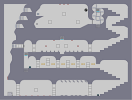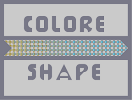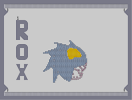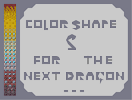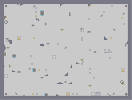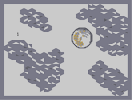Hypermodern Building Colore Shape Safrosoft's RoX Color Shape 2 Hurican Thunderbolt Silvermoon

Pages: (0)

### 4.5

Nice one. Looks more like a caterpillar to me for some reason.

### No Wait

Ive changed my mind. 4

### Dragon Art Is Getting Really Boring Now

would have been 4.5/5, but its just too common.
3.5/5

### 16 minutes?

crazy man... just crazy 5/5 i like the view on this dragon

### 5/5

With Necro; It's not your best, but very original. IMHO, stick with the 2D elements, unless you can make something crazy

But great job in taking my advice with the golden pupil! Looks awesome :)

### ....

then it would have been enough to edit the map. i thought you resubmitted because i rated it 3.5/5.

### What do u mean tl?

Yes, i resubmittet it, after 30 s. The txt was wrong in formulation.

### ?

did you resubmit after my rating?

### It's good,

but not you best...